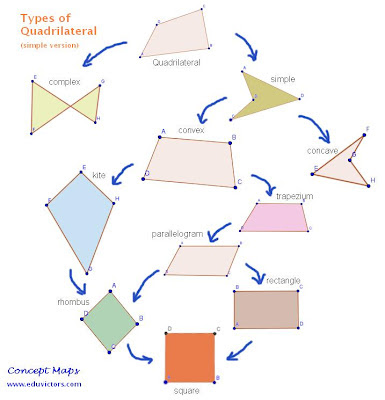Important Points to remember

Based on Class 9 NCERT Chapter on QuadrilateralsSimple Classification of Quadrilaterals. For detailed version check at wikipedia

A simple closed figure bounded by four line segments is called quadrilateral.

The sum of (interior) angles of a quadrilateral is 360°- Angle Sum Property of Quadrilateral

A quadrilateral in which one pair of opposite sides is parallel is called trapezium.

A quadrilateral in which both pairs of opposite sides are parallel is called parallelogram.

If one of the angles of a parallelogram is right angle then it is a rectangle.

If all sides of a quadrilateral are equal then it is called rhombus.

If two adjacent sides of a rectangle are equal, then it is called a square.

A quadrilateral in which two pairs of adjacent sides are equal is called a kite (or diamond.)

In a parallelogram:
ⓐ opposite sides are equal.
ⓑ opposite angles are equal.
ⓒ diagonals bisect each other.

A quadrilateral is a parallelogram, if
ⓐ opposite sides are equal.
ⓑ opposite angles are equal.
ⓒ diagonals bisect each other.
ⓓ a pair of opposite sides is equal and parallel.

Diagonals of a rhombus bisect each other at right angles and vice-versa.

Diagonals of a rectangle bisect each other and are equal and vice-versa.

Diagonals of a square bisect each other at right angles and are equal, and vice-versa.

A quadrilateral formed by joining the mid-points of the sides of a quadrilateral ,in order, is a parallelogram.

Theorems Of Parallelograms

Theorem 1: A diagonal of a parallelogram divides it into two congruent triangles. (See Proof)

Theorem 2: In a parallelogram, opposite sides are equal. (See Proof)

Theorem 3: If each pair of opposite sides of a quadrilateral is equal, then it is a parallelogram. (Proof)

Theorem 4: In a parallelogram, opposite angles are equal. (See Proof)

Theorem 5: If each pair of opposite angles of a quadrilateral is equal, then it is a parallelogram. (See Proof)

Theorem 6: If a pair of opposite sides of a quadrilateral is equal and parallel, then it is a parallelogram. (See proof)

Theorem 7: The diagonals of a parallelogram bisect each other. (See Proof)

Theorem 8: If the diagonals of a quadrilateral bisect each other, then it is a parallelogram. (See Proof)

Mid-Point Theorem

The line segment joining the mid-points of any two sides of a triangle is parallel to the third side and is equal to half of it. (see proof)

Converse of Mid-Point Theorem
The line drawn through the mid-point of one side of a triangle parallel to another side bisects the third side. (see proof)

Theorem of Intercepts
If a traversal makes equal intercepts on three or more parallel lines, then any other line cutting them also makes equal intercepts.